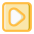# Class 9 Linear Equations In Two Variables Elimination Method

₹149

### ₹149

• #### EnglishWhat is an Equation

00:02:07Content of Simultaneous Equation

00:01:14How Eilmation Method is used to Solve Simultaneous Equation

00:03:22Master Problem 1 of Elimination Method

00:04:03Master Problem 2 of Elimination Method

00:03:54Master Problem 3 of Elimination Method

00:03:41Master Problem 1 of Equation Reducible to Lenier Equation

00:03:27Master Problem 2 of Equation Reducible to Lenier Equation

00:02:51Cost Related Word Problem 1

00:02:13Cost Related Word Problem 2

00:02:02Cost Related Word Problem 3

00:02:13Cost Related Word Problem 4

00:02:08Cost Related Word Problem 5

00:02:17

### Description

## This course will be updating soon.

Beginner

### What Student Will Learn

V1: What is an Equation V2: Content of Simultaneous Equation V3: How Eilmation Method is used to Simultaneous Equation V4: Master Problem 1 of Eilmation Method V5: Master Problem 2 of Eilmation Method V6: Master Problem 3 of Eilmation Method V7: Master Problem 1 of Equation Reducable to Lenier Equation V8: Master Problem 2 of Equation Reducable to Lenier Equation V9: Cost Related Word Problem 1 V10: Cost Related Word Problem 1 V11: Cost Related Word Problem 1 V12: Cost Related Word Problem 1 V13: Cost Related Word Problem 1

### Requirement of the Course

Maths basic till 5thRajendra KhandekarTotal Number of Course(s): 2Total Number of student(s) enrolled in all courses: 1Total Number of student(s) reviewed: 0

0.0

0

0

0

0

0